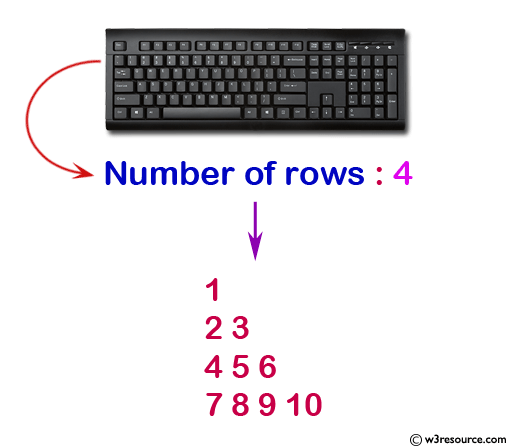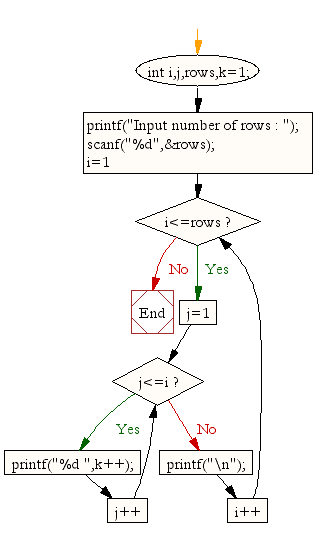﻿ C : Pattern, right angle triangle with number increased by 1

# C Exercises: Display the pattern like right angle triangle with number increased by 1

## C For Loop: Exercise-12 with Solution

Write a program in C to make such a pattern like a right angle triangle with the number increased by 1.
The pattern is as follows :

```   1
2 3
4 5 6
7 8 9 10
```

Visual Presentation:Sample Solution:

C Code:

``````#include <stdio.h>  // Include the standard input/output header file.

int main() {
int i, j, rows, k = 1;  // Declare variables 'i' and 'j' for loop counters, 'rows' for user input, 'k' for incrementing numbers.

printf("Input number of rows : ");  // Print a message to prompt user input.
scanf("%d", &rows);  // Read the value of 'rows' from the user.

for (i = 1; i <= rows; i++) {  // Start a loop to generate rows.
for (j = 1; j <= i; j++)  // Nested loop to print numbers based on the current row.
printf("%d ", k++);  // Print the value of 'k' and increment it.

printf("\n");  // Move to the next line for the next row.
}
return 0;  // Indicate that the program has executed successfully.
}
```
```

Sample Output:

```Input number of rows : 4
1
2 3
4 5 6
7 8 9 10
```

Explanation:

```for (i = 1; i <= rows; i++) {
for (j = 1; j <= i; j++)
printf("%d ", k++);
printf("\n");
}
```

In the above loop, the variable i is initialized to 1, and the loop will continue as long as i is less than or equal to the value of variable 'rows'. In each iteration of the outer loop, another loop is started with variable j, initialized to 1, and it will continue as long as j is less than or equal to the value of i.

In each iteration of the inner loop, the printf function will print the value of k to the console, followed by a space character. The value of k will be incremented by 1 in each iteration of the inner loop using the postfix increment operator (k++), so that each value printed is unique.

After the inner loop completes, the outer loop will print a newline character (\n) to create a new line.

Flowchart:C Programming Code Editor:

What is the difficulty level of this exercise?

Test your Programming skills with w3resource's quiz.

﻿